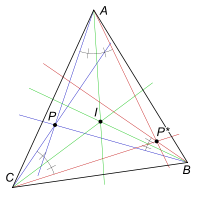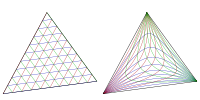# Isogonal conjugateIsogonal conjugate of P.Isogonal conjugate transformation over the points inside the triangle.

In geometry, the isogonal conjugate of a point P with respect to a triangle ABC is constructed by reflecting the lines PA, PB, and PC about the angle bisectors of A, B, and C respectively. These three reflected lines concur at the isogonal conjugate of P. (This definition applies only to points not on a sideline of triangle ABC.)

The isogonal conjugate of a point P is sometimes denoted by P*. The isogonal conjugate of P* is P.

The isogonal conjugate of the incentre I is itself. The isogonal conjugate of the orthocentre H is the circumcentre O. The isogonal conjugate of the centroid G is (by definition) the symmedian point K. The isogonal conjugates of the Fermat points are the isodynamic points and vice versa. The Brocard points are isogonal conjugates of each other.

In trilinear coordinates, if X = x : y : z is a point not on a sideline of triangle ABC, then its isogonal conjugate is 1/x : 1/y : 1/z. For this reason, the isogonal conjugate of X is sometimes denoted by X 1. The set S of triangle centers under trilinear product, defined by

(p : q : r) * (u : v : w) = pu : qv : rw,

is a commutative group, and the inverse of each X in S is X 1.

As isogonal conjugation is a function, it makes sense to speak of the isogonal conjugate of sets of points, such as lines and circles. For example, the isogonal conjugate of a line is a circumconic; specifically, an ellipse, parabola, or hyperbola according as the line intersects the circumcircle in 0, 1, or 2 points. The isogonal conjugate of the circumcircle is the line at infinity. Several well-known cubics (e.g., Thompson cubic, Darboux cubic, Neuberg cubic) are self-isogonal-conjugate, in the sense that if X is on the cubic, then X 1 is also on the cubic.